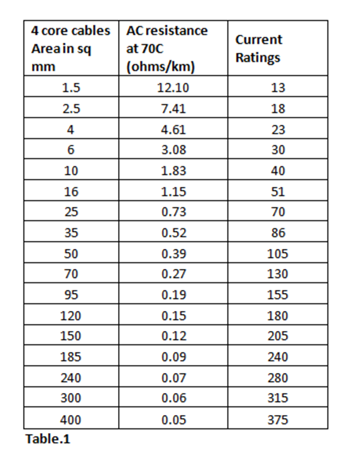Have you ever looked at your electricity bill and wondered why you are being charged for more than your consumption? This problem is often faced by industrial consumers, who are billed on kVAh supplied instead of kWh consumed. Let us first try to understand what these terms mean and what role does power factor play in your electricity consumption.

Suppose you order a glass of beer. You pay for a “full” glass of beer, but instead, what you get is a glass partially filled with froth. If the beer in your glass is kWh, full glass is kVAh, and power factor is the proportion of glass filled with beer, i.e. kWh/kVAh.

Industrial consumers or big office spaces are supplied with electricity through HT line (11kV or 33kV), unlike residential consumers. They are also charged on kVAh basis. It, thus, becomes imperative that they maintain a high power factor (as less froth in glass of beer as possible).

Let us look at some numbers to get a better idea of losses due to low power factor.

Consumer 1 and consumer 2 are both drawing 800kW of power at 0.95 and 0.9 power factors, respectively.

p1=800kW; p.f.1=0.95; t1=24 hours

p2=800kW; p.f.2=0.9; t2=24 hours

kVAh1= p1*t1/p.f.1=20,210 kVAh

kVAh2= p2*t2/p.f.2=21,333 kVAh

Although both the consumers have similar loads (similar since only their active powers were same), their energy consumption differs by 1,123 kVAh.

Additionally, a low power factor results in copper losses due to higher currents. Let us see how using the same example.

Due to small current (of the order of mA), copper losses can be neglected on HT side.

Analysis for LT side

P1=800 kW; V1=430 V; p.f.1=0.95

I1=1,958 A

P2=800 kW; V2=430 V; p.f.2=0.9

I2=2,067 AAC cable resistance and current ratings

Assuming resistance to be 0.19ohm/km (refer to Table 1) for a 50m cable, copper losses (current^2 x resistance x time) incurred in 24 hours will be:

Consumer 1: 874 kWh or 920kVAh

Consumer 2: 974 kWh or 1,082 kVAh

This amounts to a difference of 100kWh, which further accounts to a difference of 162 kVAh in billing.

Stay tuned to know the prescription for this froth on the beer.